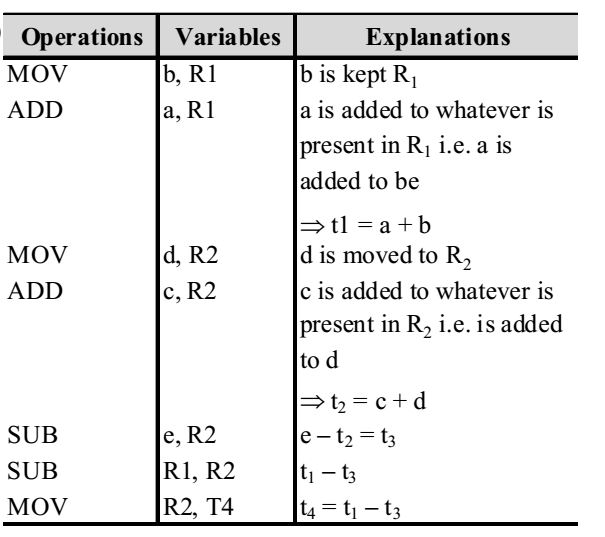## Compiler design miscellaneous

#### Compiler Design

1. Consider the following C code segment :
for (i = 0, i < n; i ++) {
for (j = 0, j < n; j ++) {
if (i % 2){
x + = (4 * j + 5 * i);
Y + = (7 + 4 * j);}}}
Which one of the following is false?

1. The expression (4*j) is used at two places- so common sub-expression elimination is possible i% 2 is loop invariant for the inner loop
5*i is also loop invariant for inner loop x + = 5*i can be replaced by x + = p;
p + = 5; (p must be initialized to 0 before the loop).
Thus replacing * with + gives strength reduction. So, only (d) is false here.

##### Correct Option: D

The expression (4*j) is used at two places- so common sub-expression elimination is possible i% 2 is loop invariant for the inner loop
5*i is also loop invariant for inner loop x + = 5*i can be replaced by x + = p;
p + = 5; (p must be initialized to 0 before the loop).
Thus replacing * with + gives strength reduction. So, only (d) is false here.

1. In a simplified computer, the instructions are
OP Rj, Ri – Performs Rj OP Ri and stores the result in register Ri .
OP m, Rj – Performs val OP Ri and stores the result in Rj. val denotes the content of memory location m.
MOV m, Ri – Moves the content of memory location m to register Ri.
MOV, Ri, m – Moves the content of the register Ri to memory location m.
The computer has only two registers, and OP is either ADD or SUB. Consider the following basic block:
t1 = a + b
t2 = c + d
t3 = e – t2
t4 = t1 – t3
Assume that all operands are initially in memory. The final value of the computation should be in memory. What is the minimum number of MOV instructions in the code generated for this basic block?

1.This how, we obtain 3 MOV instruction in the code generated for the basic block.

##### Correct Option: BThis how, we obtain 3 MOV instruction in the code generated for the basic block.

1. Some code optimizations are carried out on the intermediate code because

1. Code optimizations are carried out on the intermediate code because program analysis is more accurte on intermediate code than on machine code.

##### Correct Option: B

Code optimizations are carried out on the intermediate code because program analysis is more accurte on intermediate code than on machine code.

1. The grammar S → aSa | bS| c is

1. S → aSa | bS | C
The above grammar is LL (1) because,
First [aSa] ∩ first [bS] = (a) ∩ (b) = φ
First [bS] ∩ first [c] = (b) ∩ (c) = φ
First [c] ∩ first [aSa] = (c) ∩ (a) = φ
As the above grammar is LL (1), also LR (1) because LL (1) grammar is always LR (1) grammar.

##### Correct Option: C

S → aSa | bS | C
The above grammar is LL (1) because,
First [aSa] ∩ first [bS] = (a) ∩ (b) = φ
First [bS] ∩ first [c] = (b) ∩ (c) = φ
First [c] ∩ first [aSa] = (c) ∩ (a) = φ
As the above grammar is LL (1), also LR (1) because LL (1) grammar is always LR (1) grammar.

1. For a C program accessing X[i][j][k], the following intermediate code is generated by a compiler. Assume that the size of an integer is 32 bits and the size of a character is 8 bits.
t0 = i * 1024
t1 = j * 32
t2 = k * 4
t3 = t1 + t0
t4 = t3 + t2
t5 = X[t4]
Which one of the following statements about the source code for the C program is

1. X is declared as “int ×   ”

##### Correct Option: A

X is declared as “int ×   ”